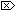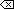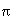The Open Group Base Specifications Issue 6
IEEE Std 1003.1-2001

#### NAME

tan, tanf, tanl - tangent function

#### SYNOPSIS

```#include <math.h> double tan(double x); float tanf(float x); long double tanl(long double x); ```

#### DESCRIPTION

[CX]The functionality described on this reference page is aligned with the ISO C standard. Any conflict between the requirements described here and the ISO C standard is unintentional. This volume of IEEE Std 1003.1-2001 defers to the ISO C standard.These functions shall compute the tangent of their argument x, measured in radians.

An application wishing to check for error situations should set errno to zero and call feclearexcept(FE_ALL_EXCEPT) before calling these functions. On return, if errno is non-zero or fetestexcept(FE_INVALID | FE_DIVBYZERO | FE_OVERFLOW | FE_UNDERFLOW) is non-zero, an error has occurred.

#### RETURN VALUE

Upon successful completion, these functions shall return the tangent of x.

If the correct value would cause underflow, and is not representable, a range error may occur, and [MX]either 0.0 (if supported), oran implementation-defined value shall be returned.

[MX]If x is NaN, a NaN shall be returned.

If x is ±0, x shall be returned.

If x is subnormal, a range error may occur and x should be returned.

If x is ±Inf, a domain error shall occur, and either a NaN (if supported), or an implementation-defined value shall be returned.

If the correct value would cause underflow, and is representable, a range error may occur and the correct value shall be returned.[XSI]If the correct value would cause overflow, a range error shall occur and tan(), tanf(), and tanl() shall return the value of the macro HUGE_VAL, HUGE_VALF, and HUGE_VALL, respectively.#### ERRORS

These functions shall fail if:

Domain Error
[MX]The value of x is ±Inf.

If the integer expression (math_errhandling & MATH_ERRNO) is non-zero, then errno shall be set to [EDOM]. If the integer expression (math_errhandling & MATH_ERREXCEPT) is non-zero, then the invalid floating-point exception shall be raised.Range Error
[XSI]The result overflows

If the integer expression (math_errhandling & MATH_ERRNO) is non-zero, then errno shall be set to [ERANGE]. If the integer expression (math_errhandling & MATH_ERREXCEPT) is non-zero, then the overflow floating-point exception shall be raised.These functions may fail if:

Range Error
The result underflows, [MX]or the value of x is subnormal.If the integer expression (math_errhandling & MATH_ERRNO) is non-zero, then errno shall be set to [ERANGE]. If the integer expression (math_errhandling & MATH_ERREXCEPT) is non-zero, then the underflow floating-point exception shall be raised.

The following sections are informative.

#### EXAMPLES

##### Taking the Tangent of a 45-Degree Angle
```#include <math.h>
...
double radians = 45.0 * M_PI / 180;
double result;
...
result = tan (radians);

```

#### APPLICATION USAGE

There are no known floating-point representations such that for a normal argument, tan( x) is either overflow or underflow.

These functions may lose accuracy when their argument is near a multiple of/2 or is far from 0.0.

On error, the expressions (math_errhandling & MATH_ERRNO) and (math_errhandling & MATH_ERREXCEPT) are independent of each other, but at least one of them must be non-zero.

None.

#### FUTURE DIRECTIONS

None.

atan() , feclearexcept() , fetestexcept() , isnan() , the Base Definitions volume of IEEE Std 1003.1-2001, Section 4.18, Treatment of Error Conditions for Mathematical Functions, <math.h>

#### CHANGE HISTORY

First released in Issue 1. Derived from Issue 1 of the SVID.

#### Issue 5

The last two paragraphs of the DESCRIPTION were included as APPLICATION USAGE notes in previous issues.

#### Issue 6

The tanf() and tanl() functions are added for alignment with the ISO/IEC 9899:1999 standard.

The DESCRIPTION, RETURN VALUE, ERRORS, and APPLICATION USAGE sections are revised to align with the ISO/IEC 9899:1999 standard.

IEC 60559:1989 standard floating-point extensions over the ISO/IEC 9899:1999 standard are marked.

End of informative text.

UNIX ® is a registered Trademark of The Open Group.
POSIX ® is a registered Trademark of The IEEE.
[ Main Index | XBD | XCU | XSH | XRAT ]